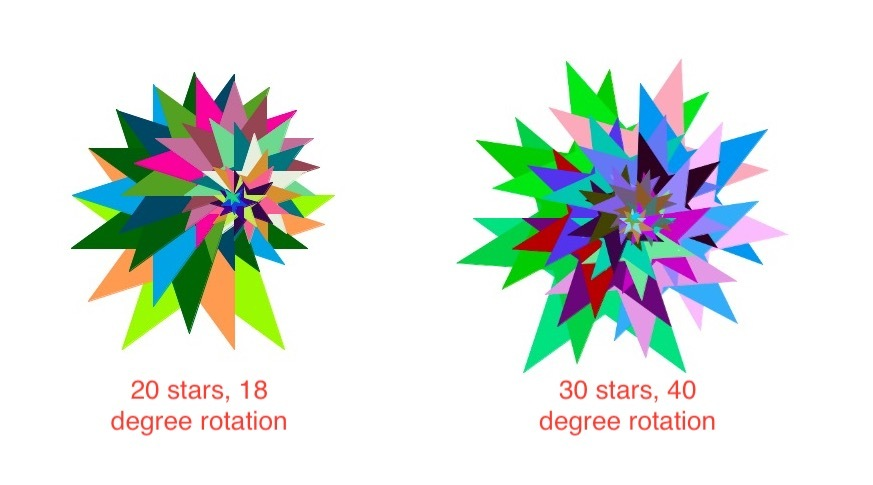GFG App
Open AppBrowser
Continue

# Draw Colourful Star Pattern in Turtle – Python

In this article we will use Python’s turtle library to draw a spiral of stars, filled with randomly generated colours. We can generate different patterns by varying some parameters.modules required:

turtle:

turtle library enables users to draw picture or shapes using commands, providing them with a virtual canvas.
turtle comes with Python’s Standard Library. It needs a version of Python with Tk support, as it uses tkinter for the graphics.

Explanation:
First we set each of the parameters for the spiral: number of stars, exterior angle of the stars and angle of rotation for the spiral. The colours are chosen randomly by choosing three random integers for rgb values, and so each time we get a different colour combination.
In the implementation below, we will draw a pattern of 30 stars, with exterior angle 144 degrees and angle of rotation 18 degrees.

## Python3

 `from` `turtle ``import` `*`   `import` `random`   `speed(speed ``=``'fastest'``)`   `def` `draw(n, x, angle):` `    ``# loop for number of stars` `    ``for` `i ``in` `range``(n):` `        `  `        ``colormode(``255``)` `        `  `        ``# choosing random integers ` `        ``# between 0 and 255` `        ``# to generate random rgb values ` `        ``a ``=` `random.randint(``0``, ``255``)` `        ``b ``=` `random.randint(``0``, ``255``)` `        ``c ``=` `random.randint(``0``, ``255``)` `        `  `        ``# setting the outline ` `        ``# and fill colour` `        ``pencolor(a, b, c)` `        ``fillcolor(a, b, c)` `        `  `        ``# begins filling the star` `        ``begin_fill()` `        `  `        ``# loop for drawing each star` `        ``for` `j ``in` `range``(``5``):` `             `  `            ``forward(``5` `*` `n``-``5` `*` `i)` `            ``right(x)` `            ``forward(``5` `*` `n``-``5` `*` `i)` `            ``right(``72` `-` `x)` `            `  `        ``# colour filling complete` `        ``end_fill()` `        `  `        ``# rotating for` `        ``# the next star` `        ``rt(angle)` `        `    `# setting the parameters` `n ``=` `30`    `# number of stars` `x ``=` `144`   `# exterior angle of each star` `angle ``=` `18`    `# angle of rotation for the spiral`   `draw(n, x, angle)`

Output:

By changing the exterior angle to 72, we can get a pattern of pentagons as such:

My Personal Notes arrow_drop_up